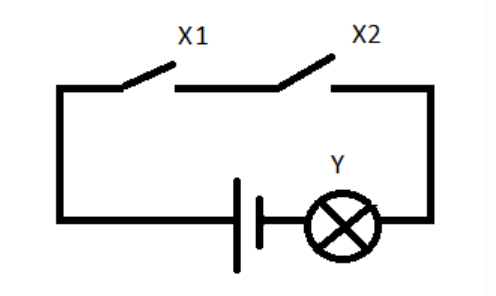# Digital Circuits Questions & Answers

1. In analytical instrumentation, little circuitry is concerned with the amplification and processing of signals.

a) True
b) False

Explanation: In analytical instrumentation, most circuitry is concerned with the amplification and processing of signals. The signals are usually in analog form.

2. Which of the following samples the analog signals to obtain their digital equivalent?

a) Analog to digital converter
b) Electronic counter
c) Comparator
d) Digital to analog converter

Explanation: Analog to digital converter samples the analog signals to obtain their digital equivalent. There are different types of analog to digital converters.

3. Which of the following can be used to store information?

a) Gates
c) DAC
d) Flip-flops

Explanation: Flip-flops can be used to store information. They are used in sequential circuits.

4. Which of the following is known as nibble?

a) 1 bit
b) 4 bits
c) 8 bits
d) 16 bits

Explanation: 4 bit units are known as nibbles. A byte consists of two nibbles.

5. Which of the following is the binary equivalent of the decimal number 10?

a) 1100
b) 1010
c) 1011
d) 1110

Explanation: The binary equivalent of the decimal number 10 is 1010. The base of binary numbers is 2.

6. 1001 is a data presented on a set of binary coded decimal output lines. What would be the decimal equivalent of this number in the case of negative logic?

a) 9
b) 6
c) 1
d) 8

Explanation: The decimal equivalent of the number 1001 in the case of negative logic is 6. In the case of positive logic, the decimal equivalent will be 9.

7. Given below is the equivalent circuit of a logic gate. Identify the logic gate.a) AND gate
b) OR gate
c) EX-OR gate
d) NOT gate

Explanation: The given circuit is the AND gate. The output of the gate is, Y=X1.X2

8. Two switches are in parallel and are connected with a lamp and a supply in series. This represents which of the following logic gate?

a) AND gate
b) OR gate
c) EX-OR gate
d) NOT gate

Explanation: Two switches are in parallel and are connected with a lamp and a supply in series. This forms the OR gate.

9. The output will be logic ‘1’ only if all the inputs are ‘0’. Which gate must be used to execute this condition?

a) AND gate
b) NAND gate
c) NOR gate
d) EX-OR gate

Explanation: The output will be logic ‘1’ only if all the inputs are ‘0’. NAND gate must be used to execute this condition.

10. INHIBIT gate is ________ gate with an inhibiting input.

a) AND gate
b) OR gate
c) EX-OR gate
d) NOT gate

Explanation: INHIBIT gate is OR gate with an inhibiting input. The gate is useful for controlling the inputs with an inhibiting signal.